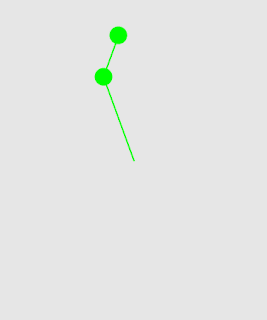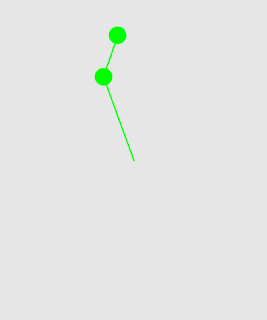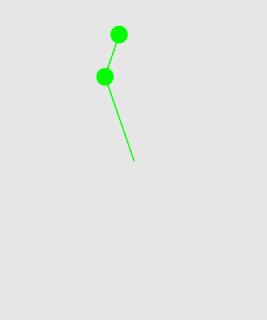## Thursday, 15 October 2015

### Simulating a Double Pendulum in Mathematica

I've been playing around with Mathematica's Non-Linear Control Systems toolbox over the past few days and it's been brilliant! One of the first systems that I tried to simulate is the double pendulum since it's such a commonly used sample problem in non-linear controls.

The first step is to write down the total kinetic and potential energies of the system and find the Lagrangian. Once you have that, you just need to run the Lagrangian through Mathematica's EulerEquations function and then set up a non-linear state space model using the differential equations.

Once you have the system model it's really simple to get the response and make a simple simulation.

And out pops a wonderful demonstration of chaos! Here are are three simulations with slightly different initial conditions showing diverging trajectories.Initial conditions: {160, -160}Initial Conditions: {161, -160}Initial Conditions: {161,-161}

The next thing I tested was whether I could make a simple controller to stabilize this double pendulum pointing straight up. I went with a standard Linear Quadratic regulator. In this simulation there is only one actuator at the elbow joint. So this is also an example of an underactuated robotic system. Works great! :D